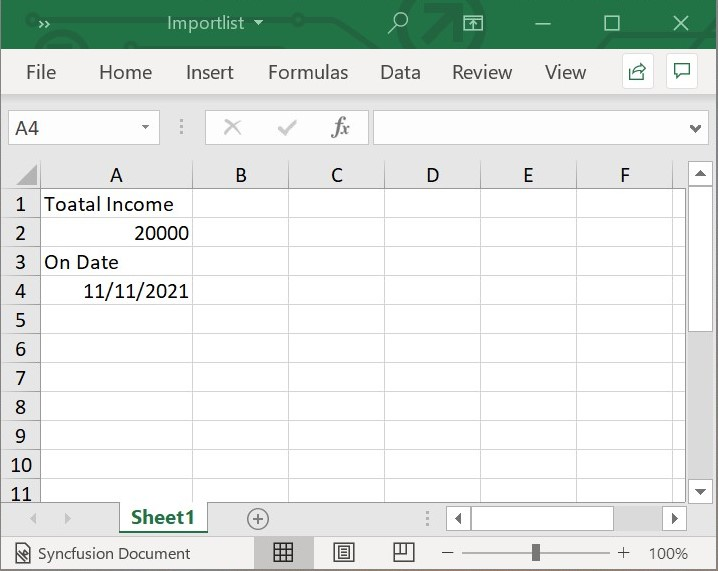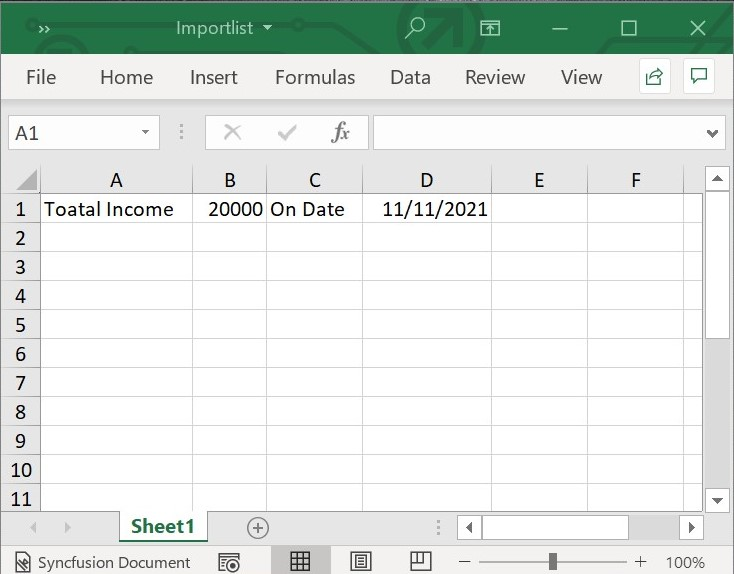# Importing Data to Worksheets

22 Apr 20212 minutes to read

Flutter XlsIO provides the ability to import data into a worksheet using List<Object>.

## Import Data from List<Object>

Using importList method, we can import list of data with different data types.

The following code snippet shows how to import a list of data vertically into the worksheet using importList() method.

• dart
• ``````// Create a new Excel Document.
final Workbook workbook = Workbook();

// Accessing sheet via index.
final Worksheet sheet = workbook.worksheets;

//Initialize the List\<Object>
final List<Object> list = [
'Toatal Income',
20000,
'On Date',
DateTime(2021, 11, 11)
];

// Represent the starting row.
final int firstRow = 1;

// Represent the starting column.
final int firstColumn = 1;

// Represents that the data should be imported vertically.
final bool isVertical = true;

//Import the Object list to Sheet
sheet.importList(list, firstRow, firstColumn, isVertical);

sheet.autoFitColumn(1);

// Save and dispose workbook.
final List<int>? bytes = workbook.saveAsStream();
File('Importlist.xlsx').writeAsBytes(bytes!);
workbook.dispose();``````

The following screenshot represents the Excel file generated by vertically importing data from a List<Object> into the Excel worksheet using the Flutter XlsIO.The following code snippet shows how to import a list of data horizontally into the worksheet using importList() method.

• dart
• ``````// Create a new Excel Document.
final Workbook workbook = Workbook();

// Accessing sheet via index.
final Worksheet sheet = workbook.worksheets;

//Initialize the List\<Object>
final List<Object> list = [
'Toatal Income',
20000,
'On Date',
DateTime(2021, 11, 11)
];

// Represent the starting row.
final int firstRow = 1;

// Represent the starting column.
final int firstColumn = 1;

// Represents that the data should be imported horizontally.
final bool isVertical = false;

//Import the Object list to Sheet
sheet.importList(list, firstRow, firstColumn, isVertical);

sheet.getRangeByIndex(1, 1, 1, 4).autoFitColumns();

// Save and dispose workbook.
final List<int>? bytes = workbook.saveAsStream();
File('Importlist.xlsx').writeAsBytes(bytes!);
workbook.dispose();``````

The following screenshot represents the Excel file generated by horizontally importing data from a List<Object> into the Excel worksheet using the Flutter XlsIO.